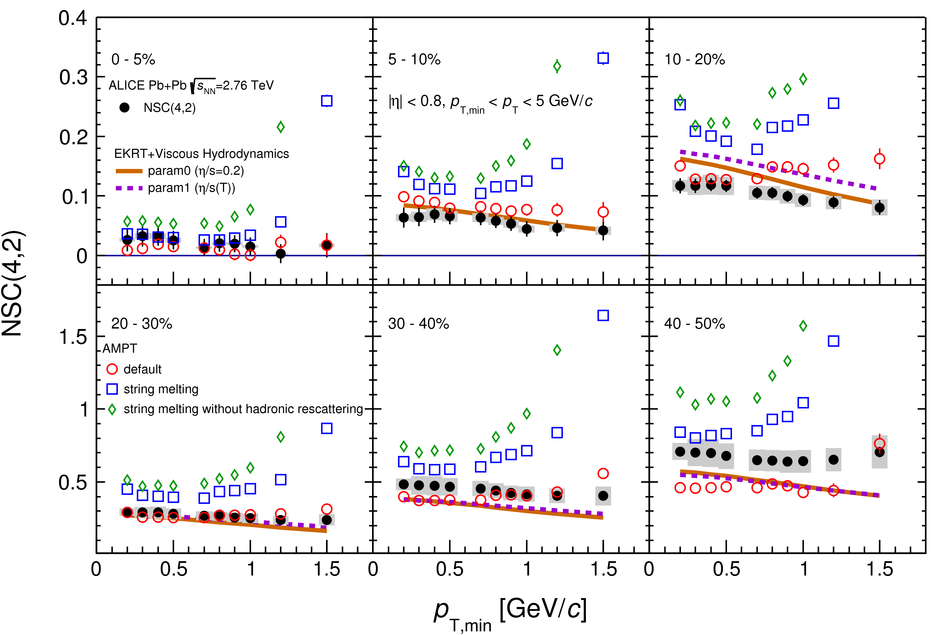Systematic studies of correlations between different order flow harmonics in Pb-Pb collisions at $\sqrt{s_{\rm NN}}$ = 2.76 TeV

The correlations between event-by-event fluctuations of anisotropic flow harmonic amplitudes have been measured in Pb-Pb collisions at $\sqrt{s_{\rm NN}}$ = 2.76 TeV with the ALICE detector at the Large Hadron Collider. The results are reported in terms of multiparticle correlation observables dubbed Symmetric Cumulants. These observables are robust against biases originating from nonflow effects. The centrality dependence of correlations between the higher order harmonics (the quadrangular $v_4$ and pentagonal $v_5$ flow) and the lower order harmonics (the elliptic $v_2$ and triangular $v_3$ flow) is presented. The transverse momentum dependences of correlations between $v_3$ and $v_2$ and between $v_4$ and $v_2$ are also reported. The results are compared to calculations from viscous hydrodynamics and A Multi-Phase Transport ({AMPT}) model calculations. The comparisons to viscous hydrodynamic models demonstrate that the different order harmonic correlations respond differently to the initial conditions and the temperature dependence of the ratio of shear viscosity to entropy density ($\eta/s$). A small average value of $\eta/s$ is favored independent of the specific choice of initial conditions in the models. The calculations with the AMPT initial conditions yield results closest to the measurements. Correlations between the magnitudes of $v_2$, $v_3$ and $v_4$ show moderate $p_{\rm T}$ dependence in mid-central collisions. This might be an indication of possible viscous corrections to the equilibrium distribution at hadronic freeze-out, which might help to understand the possible contribution of bulk viscosity in the hadronic phase of the system. Together with existing measurements of individual flow harmonics, the presented results provide further constraints on the initial conditions and the transport properties of the system produced in heavy-ion collisions.

Figures

Figure 1

 The centrality dependence of SC($m$,$n$) (a) and NSC($m$,$n$) (b) with flow harmonics for $m$ = 3--5 and $n$ = 2,3 in $\PbPb$ collisions at $\snn=2.76$~TeV. The lower order harmonic correlations [SC(3,2), SC(4,2), NSC(3,2), and NSC(4,2)] are taken from Ref.~ and shown as bands. The systematic and statistical errors are combined in quadrature for these lower order harmonic correlations. The SC(4,2) and SC(3,2) are downscaled by a factor of 0.1. Systematic uncertainties are represented with boxes for higher order harmonic correlations.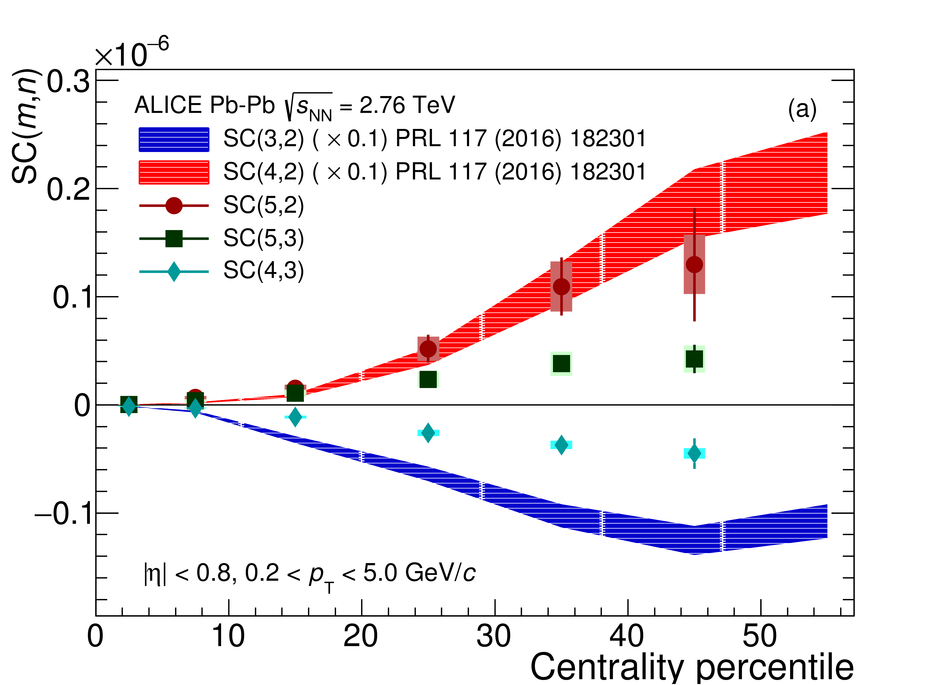Figure 2

 SC(3,2) and SC(4,2) [panels (a) and (c)] as a function of minimum $p_{\rm T}$ cuts in $\PbPb$ collisions at $\snn=2.76$~TeV are shown in the left panels. The NSC(3,2) and NSC(4,2) [panels (b) and (d)] are shown in the right panels. Systematic uncertainties are represented with boxes.Figure 3

 The individual flow harmonics $v_n$ for $n$ = 2--5 in $\PbPb$ collisions at $\snn=2.76$~TeV are shown in the left panels [(a), (b), and (c)]. $v_4$ and $v_5$ are shown in the same panel (c). The $p_{\rm T, min}$ dependence of $v_n$ for $n$ = 2--4 is shown in the right panels [(d), (e), and (f)].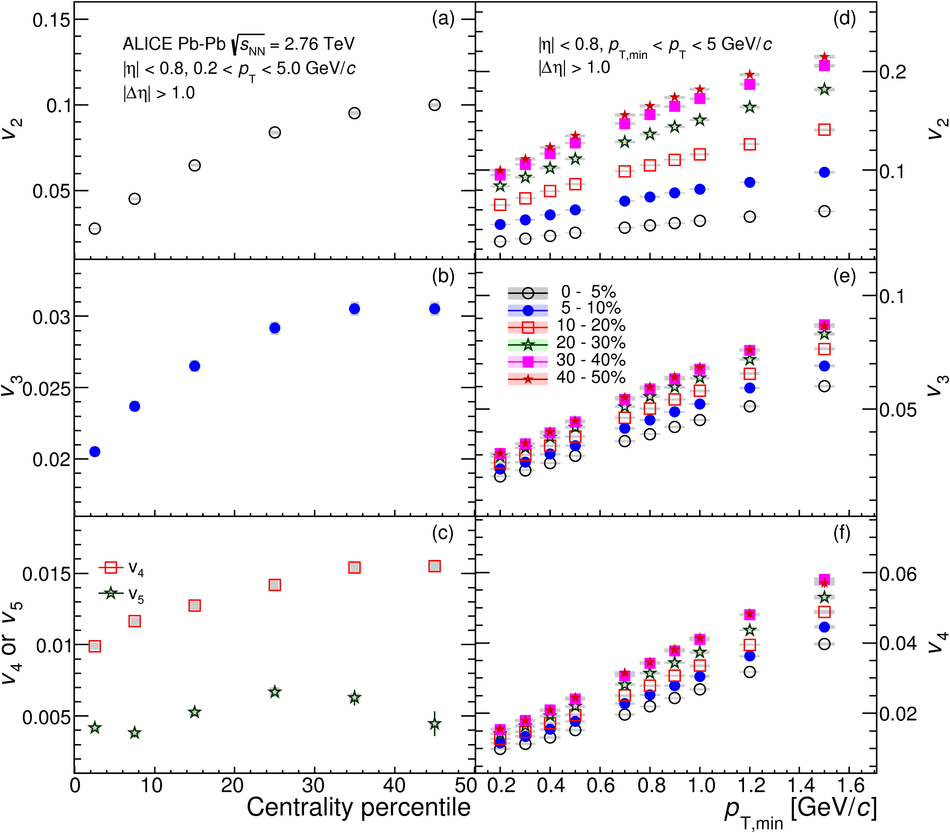Figure 4

 The centrality dependence of SC($m$,$n$) and NSC($m$,$n$) in $\PbPb$ collisions at $\snn=2.76$~TeV. Results are compared to the event-by-event EKRT+viscous hydrodynamic calculations~. The lines are hydrodynamic predictions with two different $\eta/s(T)$ parametrizations. Left (right) panels show SC($m$,$n$) (NSC($m$,$n$)).Figure 5

 The centrality dependence of SC($m$,$n$) and NSC($m$,$n$) in $\PbPb$ collisions at $\snn=2.76$~TeV. Results are compared to various VISH2+1 calculations~. Three initial conditions from AMPT, MC-KLN, and MC-Glauber are drawn as different colors and markers. The $\eta/s$ parameters are shown as different line styles, the small shear viscosity ($\eta/s=0.08$) are shown as solid lines, and large shear viscosities ($\eta/s=0.2$ for MC-KLN and MC-Glauber and 0.16 for AMPT) are drawn as dashed lines. Left (right) panels show SC($m$,$n$) (NSC($m$,$n$)).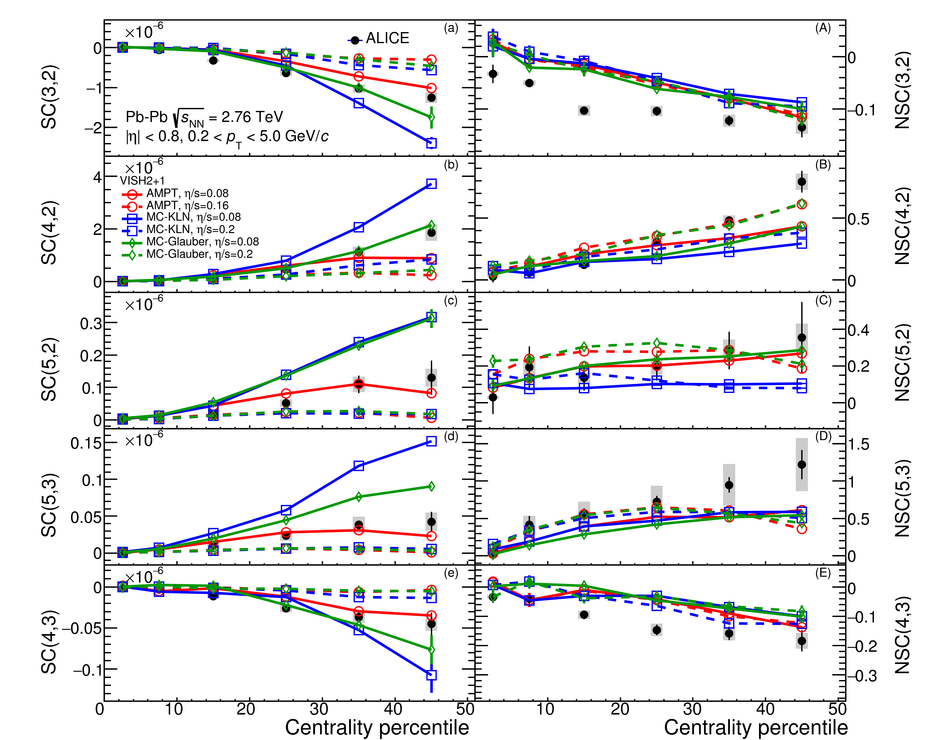Figure 6

 The centrality dependence of SC($m$,$n$) and NSC($m$,$n$) in $\PbPb$ collisions at $\snn=2.76$~TeV. Results are compared to various AMPT models. Left (right) panels show SC($m$,$n$) (NSC($m$,$n$)).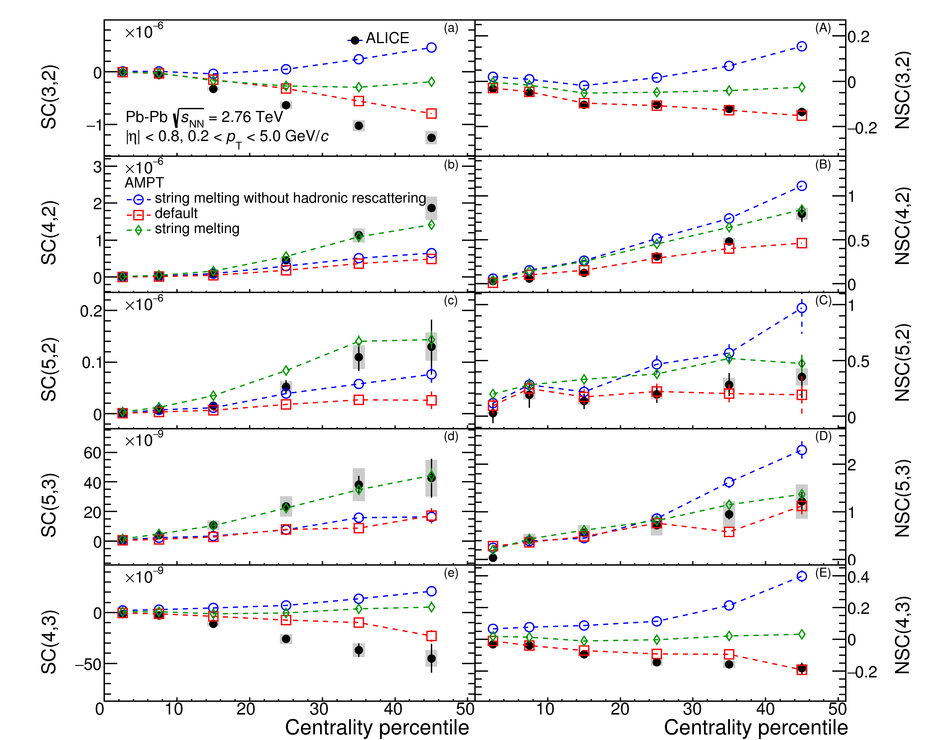Figure 7

 The $\chisqndf$ values calculated by Eq.~(\ref{Eq:chisq}) are shown for SC($m$,$n$) (a), NSC($m$,$n$) (b), and individual harmonics $v_n$ (c). Results are for model calculations which are best in describing the SC observables for each of the three different types of models.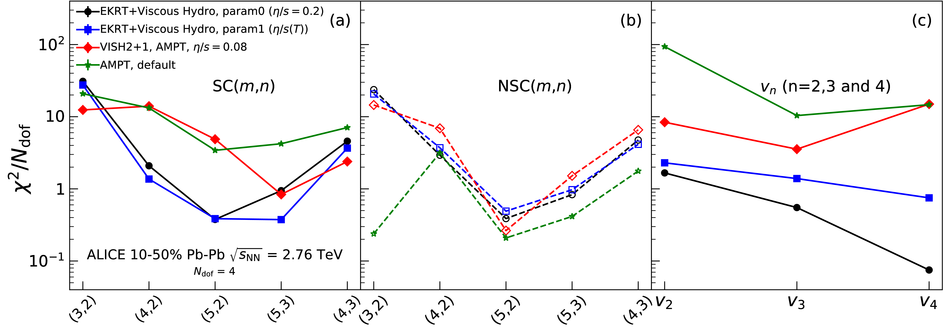Figure 8

 NSC(3,2) as a function of the minimum $p_{\rm T}$ cut in $\PbPb$ collisions at $\snn=2.76$~TeV. Results are compared to various AMPT configurations and event-by-event EKRT+viscous hydrodynamic calculations~.Figure 9

 NSC(4,2) as a function of the minimum $p_{\rm T}$ cut in $\PbPb$ collisions at $\snn=2.76$~TeV. Results are compared to various AMPT configurations and event-by-event EKRT+viscous hydrodynamic calculations~.